|

# 家电这么用,每月电费省一半!

冰箱

▼ 摆放位置很重要

冰箱尽量放置在阴凉通风处，离墙的距离最好在10厘米以上，不与音响、微波炉等电器放在一起，这些电器产生的热量会增加冰箱的耗电量。▼选择适宜温度

冷藏室调到5摄氏度，冷冻室调到零下14摄氏度最适宜，较为省电，也有利于食物储存。▼蔬果有序摆放

蔬果堆在一起会造成外冷内热，消耗电量，最好摊开摆放。水分较多的食品可用保鲜袋包好再放入冰箱，以免水分蒸发，增加除霜耗能。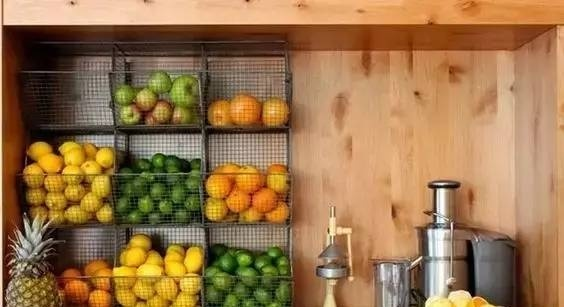▼食物储存八分满

冰箱里的食物过少，热容量会变小，增大用电量。食物过满则不利于冷空气循环，同样耗电。冰箱内部存放食物容积以80%为宜。▼在冰箱内解冻

如果冰箱里的食物需要解冻，可提前几小时将食物放到保鲜室里，这样食物的冷气能减少冰箱压缩机的运转，保鲜室内的相对温差也能使食物解冻。▼经常除霜

霜是冷的不良导体，冰箱结霜后成制冷能力下降，为冰箱定期除霜则能降低消耗，节省电量。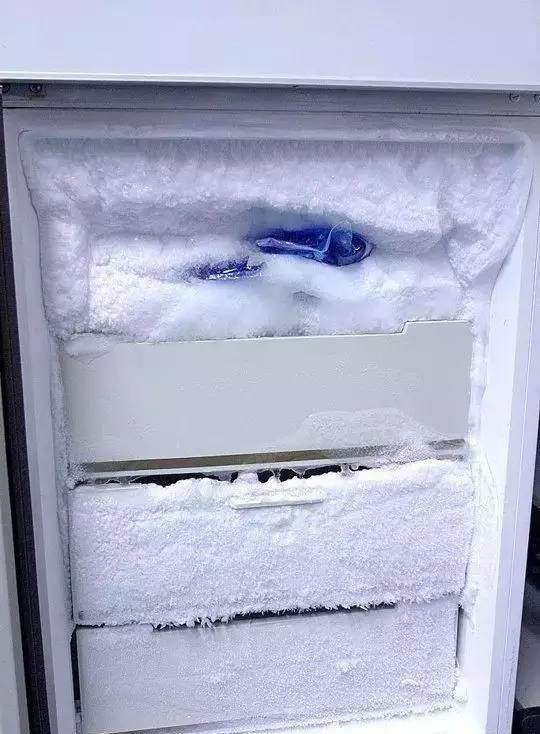空调

▼ 26℃为最佳温度

理论上，空调温度每调高一度就可以节约大约10%的电能。但考虑舒适度和健康因素，空调数字宜设置在26℃左右，这样既凉爽又不费电。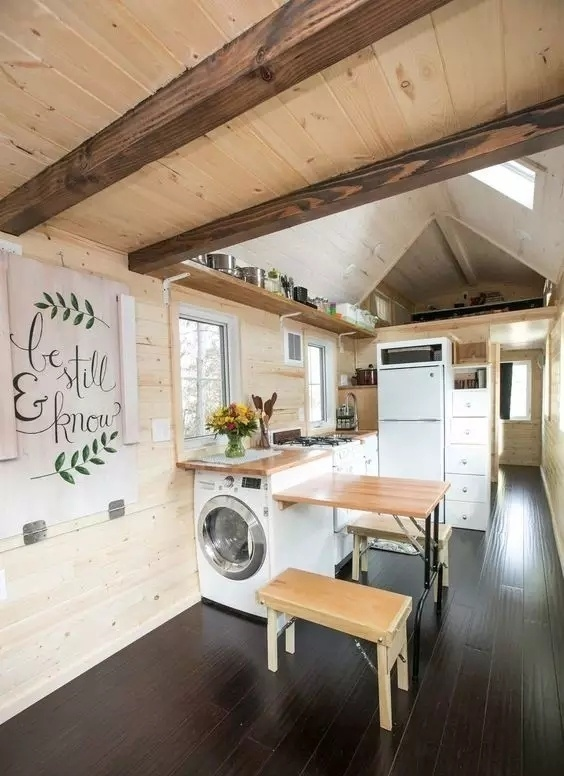▼ 提前换气少开窗

在确定要打开空调前就将房间的空气换好，如果遇到使用中一定要开门开窗的情况，建议缝隙要小、时间要短。▼ 切忌频繁开关空调

频繁开关空调会使其一直处于运作状态，更加费电。所以当室内温度降低后别急着关空调，将温度调高，风量调小就好。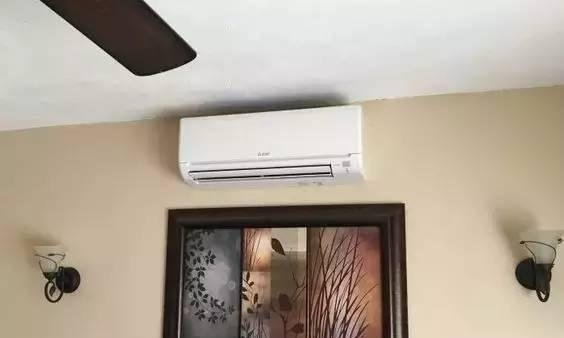▼ 开启自动模式

空调从启动到感觉凉爽这段时间最为耗电；而待室温与设定温度相同后，耗电量会降低。自动模式会依照房间状况，选择最为适当的风量及风向。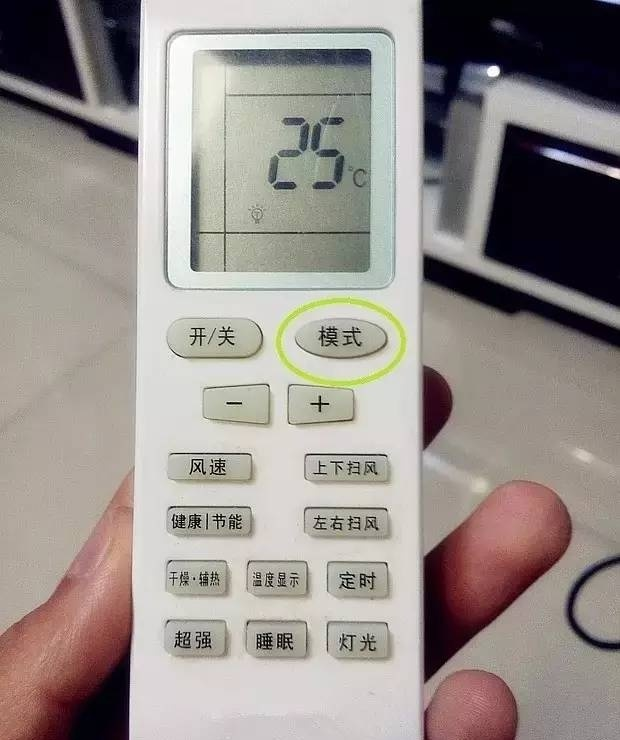▼ 巧用睡眠和定时功能

睡眠模式不仅可以让人获得舒适睡眠，还可以省电20％以上。而将空调设在凌晨4点左右定时关闭，利用余温刚好享受到睡醒，经济实用。▼ 空调外机避开阳光

空调外机不宜安装在阳光直射的地方，会影响自身的散热效果。如果只能装在向阳的一面，建议装上遮阳篷。▼ 定期清扫过滤网

空调的过滤网应隔一段时间检查并清扫，这样可以使空调的送风通畅，降低能耗，利于健康。电视机

▼ 调低音量与亮度

电视机音量每增加1瓦音频功率，就要增加3~4瓦电耗。屏幕亮度的最亮状态比最暗状态要多耗电50%~60%。使用时可将电视亮度调低，声音调小，一般可节电约10%。▼ 及时关闭电源

不关闭电源时电视的待机功耗为8.1瓦，长年累月则是一笔不小的数字。使用电器后要养成随手关闭电源的习惯，节约从点滴做起。▼加盖防尘罩

电视机如果积累很多灰尘，会增加电量消耗、影响图像和音质，还会对人体的健康产生负面影响。应给电视机加盖防尘罩。▼ 不要直接拔插电源

开关机时直接插进或拔出电源插头，会使电路时断时续，损坏电视机内部组件，损耗电量。正确做法是：开机时先插上电源插头，再开开关；关机时先关开关再拔插头。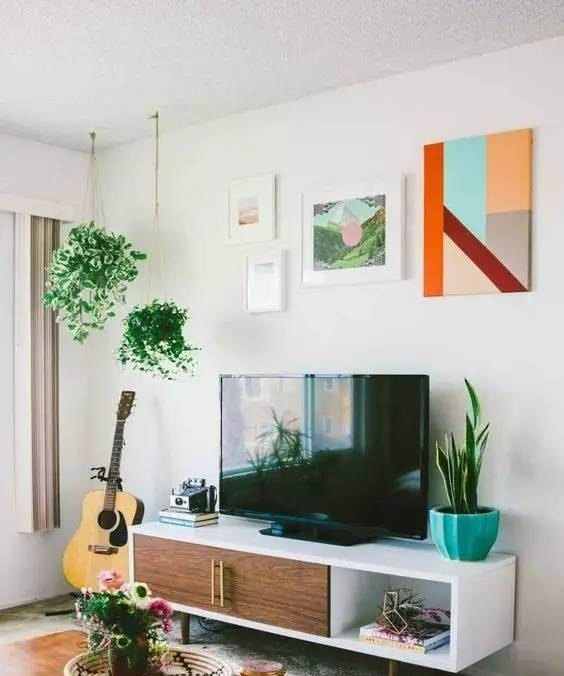洗衣机

▼ 先浸再洗

洗涤前，将衣物在洗衣液中浸泡10分钟，让洗涤剂与衣服上的污垢溶解后再洗涤，可使洗衣机的运转时间缩短一半左右，电耗也就相应减少。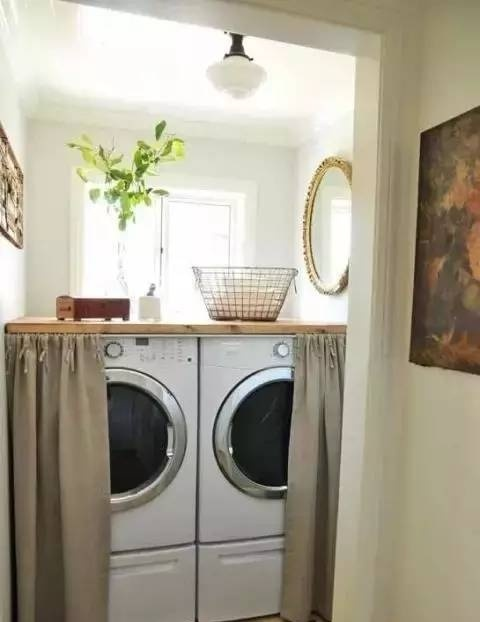▼ 使用强档洗涤

在同等洗涤时间内，采用弱档工作时电动机启动次数多，十分费电。使用强档不但能省电，还可以延长洗衣机寿命。▼ 控制脱水时间

普通涡轮式洗衣机一分钟脱水率可达55%，一般脱水不应超过3分钟，再延长脱水时间，其实意义不大。电饭煲

▼ 按人数选购电饭煲

电饭煲并不是功率小就省电，蒸同量的米饭，700瓦的电饭煲比500瓦的节省时间。因此，要考虑家庭成员因素，选择合适瓦数的电饭煲。▼ 米饭煮前先浸泡

米饭烹煮前先浸泡半小时，可以缩短电饭煲的烹煮时间，极大地减少电量消耗。利用电饭煲断电后的余热效应还能进行饭菜食物的加热。▼ 及时拔下电源插头

电饭煲用完后若不需要长时间保温，一定要拔下电源插头，否则当锅内温度下降到70度以下时，它会自动通电，既耗电又缩短使用寿命。`声明：本文由入驻焦点开放平台的作者撰写，除焦点官方账号外，观点仅代表作者本人，不代表焦点立场错误信息举报电话： 400-099-0099，邮箱：jubao@vip.sohu.com，或点此进行意见反馈，或点此进行举报投诉。`A B C D E F G H J K L M N P Q R S T W X Y Z
A - B - C - D - E
• A
• 鞍山
• 安庆
• 安阳
• 安顺
• 安康
• 澳门
• B
• 北京
• 保定
• 包头
• 巴彦淖尔
• 本溪
• 蚌埠
• 亳州
• 滨州
• 北海
• 百色
• 巴中
• 毕节
• 保山
• 宝鸡
• 白银
• 巴州
• C
• 承德
• 沧州
• 长治
• 赤峰
• 朝阳
• 长春
• 常州
• 滁州
• 池州
• 长沙
• 常德
• 郴州
• 潮州
• 崇左
• 重庆
• 成都
• 楚雄
• 昌都
• 慈溪
• 常熟
• D
• 大同
• 大连
• 丹东
• 大庆
• 东营
• 德州
• 东莞
• 德阳
• 达州
• 大理
• 德宏
• 定西
• 儋州
• 东平
• E
• 鄂尔多斯
• 鄂州
• 恩施
F - G - H - I - J
• F
• 抚顺
• 阜新
• 阜阳
• 福州
• 抚州
• 佛山
• 防城港
• G
• 赣州
• 广州
• 桂林
• 贵港
• 广元
• 广安
• 贵阳
• 固原
• H
• 邯郸
• 衡水
• 呼和浩特
• 呼伦贝尔
• 葫芦岛
• 哈尔滨
• 黑河
• 淮安
• 杭州
• 湖州
• 合肥
• 淮南
• 淮北
• 黄山
• 菏泽
• 鹤壁
• 黄石
• 黄冈
• 衡阳
• 怀化
• 惠州
• 河源
• 贺州
• 河池
• 海口
• 红河
• 汉中
• 海东
• 怀来
• I
• J
• 晋中
• 锦州
• 吉林
• 鸡西
• 佳木斯
• 嘉兴
• 金华
• 景德镇
• 九江
• 吉安
• 济南
• 济宁
• 焦作
• 荆门
• 荆州
• 江门
• 揭阳
• 金昌
• 酒泉
• 嘉峪关
K - L - M - N - P
• K
• 开封
• 昆明
• 昆山
• L
• 廊坊
• 临汾
• 辽阳
• 连云港
• 丽水
• 六安
• 龙岩
• 莱芜
• 临沂
• 聊城
• 洛阳
• 漯河
• 娄底
• 柳州
• 来宾
• 泸州
• 乐山
• 六盘水
• 丽江
• 临沧
• 拉萨
• 林芝
• 兰州
• 陇南
• M
• 牡丹江
• 马鞍山
• 茂名
• 梅州
• 绵阳
• 眉山
• N
• 南京
• 南通
• 宁波
• 南平
• 宁德
• 南昌
• 南阳
• 南宁
• 内江
• 南充
• P
• 盘锦
• 莆田
• 平顶山
• 濮阳
• 攀枝花
• 普洱
• 平凉
Q - R - S - T - W
• Q
• 秦皇岛
• 齐齐哈尔
• 衢州
• 泉州
• 青岛
• 清远
• 钦州
• 黔南
• 曲靖
• 庆阳
• R
• 日照
• 日喀则
• S
• 石家庄
• 沈阳
• 双鸭山
• 绥化
• 上海
• 苏州
• 宿迁
• 绍兴
• 宿州
• 三明
• 上饶
• 三门峡
• 商丘
• 十堰
• 随州
• 邵阳
• 韶关
• 深圳
• 汕头
• 汕尾
• 三亚
• 三沙
• 遂宁
• 山南
• 商洛
• 石嘴山
• T
• 天津
• 唐山
• 太原
• 通辽
• 铁岭
• 泰州
• 台州
• 铜陵
• 泰安
• 铜仁
• 铜川
• 天水
• 天门
• W
• 乌海
• 乌兰察布
• 无锡
• 温州
• 芜湖
• 潍坊
• 威海
• 武汉
• 梧州
• 渭南
• 武威
• 吴忠
• 乌鲁木齐
X - Y - Z
• X
• 邢台
• 徐州
• 宣城
• 厦门
• 新乡
• 许昌
• 信阳
• 襄阳
• 孝感
• 咸宁
• 湘潭
• 湘西
• 西双版纳
• 西安
• 咸阳
• 西宁
• 仙桃
• 西昌
• Y
• 运城
• 营口
• 盐城
• 扬州
• 鹰潭
• 宜春
• 烟台
• 宜昌
• 岳阳
• 益阳
• 永州
• 阳江
• 云浮
• 玉林
• 宜宾
• 雅安
• 玉溪
• 延安
• 榆林
• 银川
• Z
• 张家口
• 镇江
• 舟山
• 漳州
• 淄博
• 枣庄
• 郑州
• 周口
• 驻马店
• 株洲
• 张家界
• 珠海
• 湛江
• 肇庆
• 中山
• 自贡
• 资阳
• 遵义
• 昭通
• 张掖
• 中卫

1室1厅1厨1卫1阳台

1
2
3
4
5

0
1
2

1

1

0
1
2
3报名成功，资料已提交审核A B C D E F G H J K L M N P Q R S T W X Y Z
A - B - C - D - E
• A
• 鞍山
• 安庆
• 安阳
• 安顺
• 安康
• 澳门
• B
• 北京
• 保定
• 包头
• 巴彦淖尔
• 本溪
• 蚌埠
• 亳州
• 滨州
• 北海
• 百色
• 巴中
• 毕节
• 保山
• 宝鸡
• 白银
• 巴州
• C
• 承德
• 沧州
• 长治
• 赤峰
• 朝阳
• 长春
• 常州
• 滁州
• 池州
• 长沙
• 常德
• 郴州
• 潮州
• 崇左
• 重庆
• 成都
• 楚雄
• 昌都
• 慈溪
• 常熟
• D
• 大同
• 大连
• 丹东
• 大庆
• 东营
• 德州
• 东莞
• 德阳
• 达州
• 大理
• 德宏
• 定西
• 儋州
• 东平
• E
• 鄂尔多斯
• 鄂州
• 恩施
F - G - H - I - J
• F
• 抚顺
• 阜新
• 阜阳
• 福州
• 抚州
• 佛山
• 防城港
• G
• 赣州
• 广州
• 桂林
• 贵港
• 广元
• 广安
• 贵阳
• 固原
• H
• 邯郸
• 衡水
• 呼和浩特
• 呼伦贝尔
• 葫芦岛
• 哈尔滨
• 黑河
• 淮安
• 杭州
• 湖州
• 合肥
• 淮南
• 淮北
• 黄山
• 菏泽
• 鹤壁
• 黄石
• 黄冈
• 衡阳
• 怀化
• 惠州
• 河源
• 贺州
• 河池
• 海口
• 红河
• 汉中
• 海东
• 怀来
• I
• J
• 晋中
• 锦州
• 吉林
• 鸡西
• 佳木斯
• 嘉兴
• 金华
• 景德镇
• 九江
• 吉安
• 济南
• 济宁
• 焦作
• 荆门
• 荆州
• 江门
• 揭阳
• 金昌
• 酒泉
• 嘉峪关
K - L - M - N - P
• K
• 开封
• 昆明
• 昆山
• L
• 廊坊
• 临汾
• 辽阳
• 连云港
• 丽水
• 六安
• 龙岩
• 莱芜
• 临沂
• 聊城
• 洛阳
• 漯河
• 娄底
• 柳州
• 来宾
• 泸州
• 乐山
• 六盘水
• 丽江
• 临沧
• 拉萨
• 林芝
• 兰州
• 陇南
• M
• 牡丹江
• 马鞍山
• 茂名
• 梅州
• 绵阳
• 眉山
• N
• 南京
• 南通
• 宁波
• 南平
• 宁德
• 南昌
• 南阳
• 南宁
• 内江
• 南充
• P
• 盘锦
• 莆田
• 平顶山
• 濮阳
• 攀枝花
• 普洱
• 平凉
Q - R - S - T - W
• Q
• 秦皇岛
• 齐齐哈尔
• 衢州
• 泉州
• 青岛
• 清远
• 钦州
• 黔南
• 曲靖
• 庆阳
• R
• 日照
• 日喀则
• S
• 石家庄
• 沈阳
• 双鸭山
• 绥化
• 上海
• 苏州
• 宿迁
• 绍兴
• 宿州
• 三明
• 上饶
• 三门峡
• 商丘
• 十堰
• 随州
• 邵阳
• 韶关
• 深圳
• 汕头
• 汕尾
• 三亚
• 三沙
• 遂宁
• 山南
• 商洛
• 石嘴山
• T
• 天津
• 唐山
• 太原
• 通辽
• 铁岭
• 泰州
• 台州
• 铜陵
• 泰安
• 铜仁
• 铜川
• 天水
• 天门
• W
• 乌海
• 乌兰察布
• 无锡
• 温州
• 芜湖
• 潍坊
• 威海
• 武汉
• 梧州
• 渭南
• 武威
• 吴忠
• 乌鲁木齐
X - Y - Z
• X
• 邢台
• 徐州
• 宣城
• 厦门
• 新乡
• 许昌
• 信阳
• 襄阳
• 孝感
• 咸宁
• 湘潭
• 湘西
• 西双版纳
• 西安
• 咸阳
• 西宁
• 仙桃
• 西昌
• Y
• 运城
• 营口
• 盐城
• 扬州
• 鹰潭
• 宜春
• 烟台
• 宜昌
• 岳阳
• 益阳
• 永州
• 阳江
• 云浮
• 玉林
• 宜宾
• 雅安
• 玉溪
• 延安
• 榆林
• 银川
• Z
• 张家口
• 镇江
• 舟山
• 漳州
• 淄博
• 枣庄
• 郑州
• 周口
• 驻马店
• 株洲
• 张家界
• 珠海
• 湛江
• 肇庆
• 中山
• 自贡
• 资阳
• 遵义
• 昭通
• 张掖
• 中卫• 手机• 分享
• 设计
免费设计
• 计算器
装修计算器
• 入驻
合作入驻
• 联系
联系我们
• 置顶
返回顶部